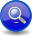Home Libraries People FAQ More

### RExpressions - Recursive ASTs!

In this example, we'll explore more on how to create heierarchical ASTs. We will parse a minimalistic JSON-like language and compile the results into our data structures in the form of a tree.

rexpr is a parser for RExpressions, a language resembling a minimal subset of json, limited to a dictionary (composed of key=value pairs) where the value can itself be a string or a recursive dictionary.

Here's an Example:

```{
"color" = "blue"
"size" = "29 cm."
"position" = {
"x" = "123"
"y" = "456"
}
}
```

This simple parser for X3 is intended as a minimal starting point. It is minimal, yet complete with all things necessary parts that make up rules (and grammars), except error handling and reporting.

The example can be found here: rexpr.cpp

The same parser, but complete with error handling and reporting, better file organization, separate compilation of grammars, and a full test harness, can be found in this directory:

Noterexpr_full is the canonical structure proposed as best practice on how parsers are written using Spirit X3.
##### The AST

Here's the AST:

```namespace client { namespace ast
{
namespace x3 = boost::spirit::x3;

struct rexpr;

struct rexpr_value : x3::variant<
std::string
, x3::forward_ast<rexpr>
>
{
using base_type::base_type;
using base_type::operator=;
};

typedef std::map<std::string, rexpr_value> rexpr_map;
typedef std::pair<std::string, rexpr_value> rexpr_key_value;

struct rexpr
{
rexpr_map entries;
};
}}
```

`x3::variant` is a support utility in Spirit X3 that extends Boost.Variant. Typically, you use Boost.Variant right out of the box and refer to a particular template instantiation using a typedef. For example:

```typedef boost::variant<std::string, int> my_variant;
```

Instead of doing that, we create a `class` (or `struct` in the case) that subclasses from `x3::variant`. By making the variant a subclass, you have a distinct type in your namespace. You also have control of the constructors and assignment operators, as well as giving you the freedom to add more functionality.

`rexpr_value` has two variant elements. It can either be a `std::string` or a `rexpr`. Since `rexpr` recursively contains a `rexpr_value`, it has to be forward declared. This recursive data structure requires `rexpr` to be wrapped in a `x3::forward_ast` as shown in the declaration.

We need to tell fusion about our `rexpr` struct to make it a first-class fusion citizen:

```BOOST_FUSION_ADAPT_STRUCT(
client::ast::rexpr,
entries
)
```

So essentially, a `rexpr_value` value is either a `std::string` or a `std::map<std::string, rexpr_value>`.

##### Walking the AST

We add a utility to print out the AST:

```int const tabsize = 4;

struct rexpr_printer
{
typedef void result_type;

rexpr_printer(int indent = 0)
: indent(indent) {}

void operator()(rexpr const& ast) const
{
std::cout << '{' << std::endl;
for (auto const& entry : ast.entries)
{
tab(indent+tabsize);
std::cout << '"' << entry.first << "\" = ";
boost::apply_visitor(rexpr_printer(indent+tabsize), entry.second);
}
tab(indent);
std::cout << '}' << std::endl;
}

void operator()(std::string const& text) const
{
std::cout << '"' << text << '"' << std::endl;
}

void tab(int spaces) const
{
for (int i = 0; i < spaces; ++i)
std::cout << ' ';
}

int indent;
};
```

Traversing the AST is a recursive exercise. `rexpr_printer` is a function object and the main entry point is ```void operator()(rexpr const& ast)```. Notice how it recursively calls an instance of itself for each of the key value pairs, using `boost::apply_visitor`. Before and after iterating through all the elements in the map, the braces are printed to surround the entire body, taking care of correct indentation at each point in the recursive traversal.

The `operator()` for `std::string` should be self explanatory. It simply prints the text inside double quotes.

##### The Grammar
```namespace client { namespace parser
{
namespace x3 = boost::spirit::x3;
namespace ascii = boost::spirit::x3::ascii;

using x3::lit;
using x3::lexeme;

using ascii::char_;
using ascii::string;

x3::rule<class rexpr_value, ast::rexpr_value>
rexpr_value = "rexpr_value";

x3::rule<class rexpr, ast::rexpr>
rexpr = "rexpr";

x3::rule<class rexpr_key_value, ast::rexpr_key_value>
rexpr_key_value = "rexpr_key_value";

auto const quoted_string =
lexeme['"' >> *(char_ - '"') >> '"'];

auto const rexpr_value_def =
quoted_string | rexpr;

auto const rexpr_key_value_def =
quoted_string >> '=' >> rexpr_value;

auto const rexpr_def =
'{' >> *rexpr_key_value >> '}';

BOOST_SPIRIT_DEFINE(rexpr_value, rexpr, rexpr_key_value);
}}
```

We declare three rules `rexpr_value`, `rexpr` and `rexpr_key_value`. Same deal as before. The attributes declared for each rule are the same structures we declared in our AST above. These are the structures our rules will produce.

So, going top-down (from the start rule), `rexpr` is defined as zero or more `rexpr_key_value`s enclosed inside the curly braces. That's the kleene star in action there: `*rexpr_key_value`.

NoteTake note of the convention: we use `rexpr` name as the rule name and `rexpr_def` as the rule definition name.

To make the grammar clean, we capture the `quoted_string` production using C++ `auto`.

`rexpr_value` is a `quoted_string` followed by the assignment operator `'='`, followed by a `rexpr_value`. `rexpr_value` is a `quoted_string` OR a `rexpr`. This uses the alternative operator `|`, and maps nicely to the variant AST the rule is associated with.

Finally, we associate the rules and their definitions:

```BOOST_SPIRIT_DEFINE(rexpr_value, rexpr, rexpr_key_value);
```